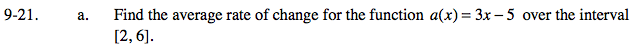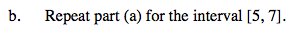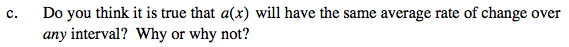### Home > PCT > Chapter Ch9 > Lesson 9.1.2 > Problem9-21

9-21.
1. Complete the following. Homework Help ✎

1. Find the average rate of change for the function a(x) = 3x − 5 over the interval [2, 6].

2. Repeat part (a) for the interval [5, 7].

3. Do you think it is true that a(x) will have the same average rate of change over any interval? Why or why not?$\frac{a(6)-a(2)}{6-2}$Think about the graph of y = a(x).
What type of curve is it?